Resampling Methods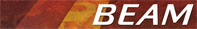### Resampling Methods

If a product is projected it comes up that the pixel centers of the target product generally not correspond to the centers of the pixels of the input product. Resampling entitles the process of determination and interpolation of pixels in the source product for computation of the pixel values in the target product. The effects of resampling will especially be visible if the pixels in the target product are larger than the source pixels.
BEAM provides three different resampling methods for this computation.

#### Nearest Neighbour

Every pixel value in th output product is set to the nearest input pixel value.
Pros Cons
Very simple, fast Some pixels get lost and others are duplicated
No new values are calculated by interpolation Loss of sharpness
Fast, compared to Cubic Convolution resampling

Following figure demonstrates the calculation of the new pixel value.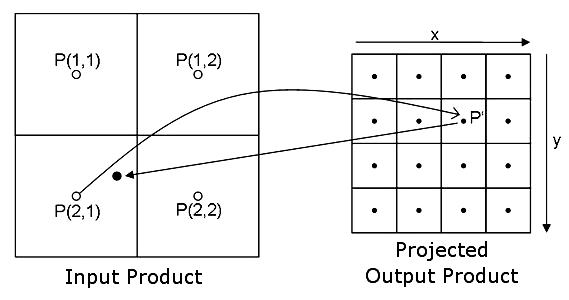#### Bi-linear Interpolation

Calculation of the new pixel value is performed by the weight of the four surrounding pixels.
ProsCons
Extremas are balanced Less contrast compared to Nearest Neighbour
Image losses sharpness compared to Nearest Neighbour New values are calculated which are not present in the input product

Following figure demonstrates the calculation of the new pixel value.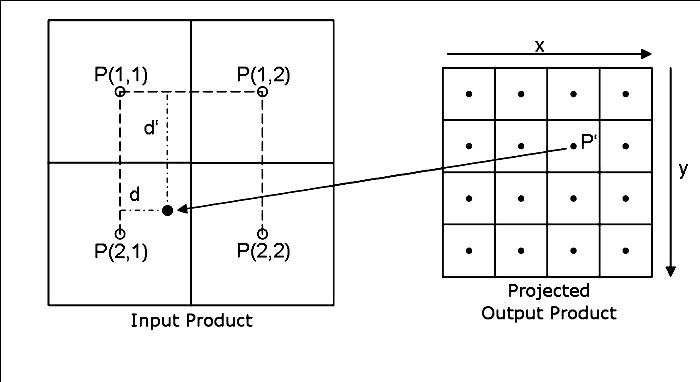The bilinear interpolation is performed by the following equation:#### Cubic Convolution

Calculation of the new pixel value is performed by weighting the 16 surrounding pixels.
Pros Cons
Extremas are balanced Less contrast compared to Nearest Neighbour
Image is sharper compared to Bi-linear Interpolation New values are calculated which are not present in the input product
Slow, compared to Nearest Neighbour resampling

Following figure demonstrates the calculation of the new pixel value.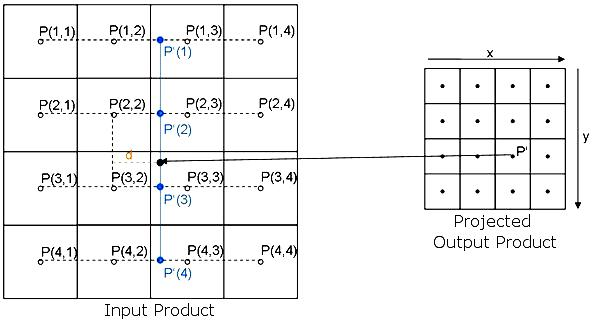The bilinear interpolation is performed by the following equation: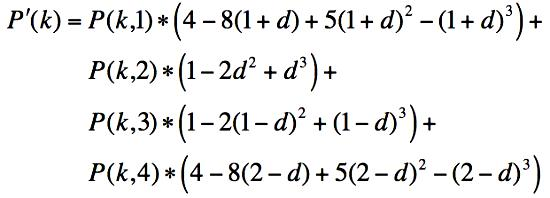In the first step the average value for each line is calculated, afterwards the new pixel value is calculated with the four new average values P'(1) - P'(4) similar to the preceding calculation.

#### Visual Comparison of the Resampling Methods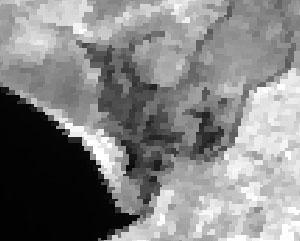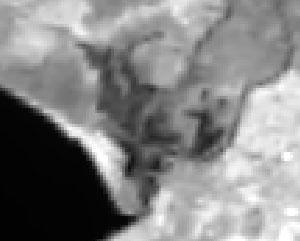Nearest Neighbour Bi-linear Interpolation Cubic Convolution